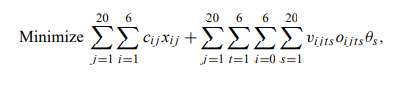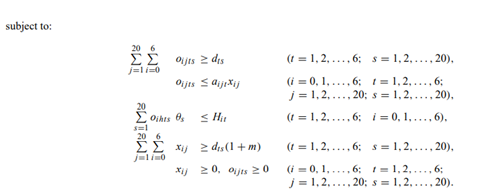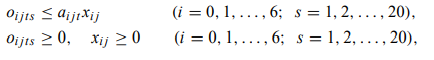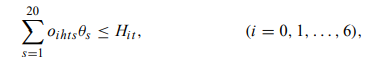# To plan for long-range energy needs, a federal agency is modeling electrical-power investments as a linear program.

To plan for long-range energy needs, a federal agency is modeling electrical-power investments as a linear program. The agency has divided its time horizon of 30 years into six periods t = 1, 2, . . . , 6, of five years each. By the end of each of these intervals, the government can construct a number of plants (hydro, fossil, gas turbine, nuclear, and so forth). Let xi j denote the capacity of plant j when initiated at the end of interval i, with per-unit construction cost of ci j . Quantities x0 j denote capacities of plants currently in use.

Given the decisions xi j on plant capacity, the agency must decide how to operate the plants to meet energy needs. Since these decisions require more detailed information to account for seasonal variations in energy demand, the agency has further divided each of the time intervals t = 1, 2, . . . , 6 into 20 subintervals s = 1, 2, . . . , 20. The agency has estimated the electrical demand in each (interval t, subinterval s) combination as dts. Let oi jts denote the operating level during the time period ts of plant j that has been constructed in interval i. The plants must be used to meet demand requirements and incur per-unit operating costs of vi jts. Because of operating limitations and aging, the plants cannot always operate at full construction capacity. Let ai jt denote the availability during time period t of plant j that was constructed in time interval i.

Typically, the coefficient ai jt will be about 0.9. Note that ai jt = 0 for t ≤ i, since the plant is not available until after the end of its construction interval i. To model uncertainties in its demand forecasts, the agency will further constrain its construction decisions by introducing a margin m of reserve capacity; in each period the total operating capacity from all plants must be at least as large as dts(1 + m).

Finally, the total output of hydroelectric power in any time interval t cannot exceed the capacity Hit imposed by availability of water sources. (In a more elaborate model, we might incorporate Hit as a decision variable.)

The linear-programming model developed by the agency is:In this formulation, θs denotes the length of time period s; the values of xi j are given. The subscript h denotes hydroelectric.

a) Interpret the objective function and each of the constraints in this model. How large is the model?

b) What is the structure of the constraint coefficients for this problem?

c) Suppose that we apply the decomposition algorithm to solve this problem; for each plant j and time period t, let the constraintsform a subproblem. What is the objective function to the subproblem at each step? Show that each subproblem either solves at oi jts = 0 and xi j = 0 for all i and s, or is unbounded. Specify the steps for applying the decomposition algorithm with this choice of subproblems.

d) How would the application of decomposition discussed in part (c) change if the constraintsare added to each subproblem in which j = h denotes a hydroelectric plant?